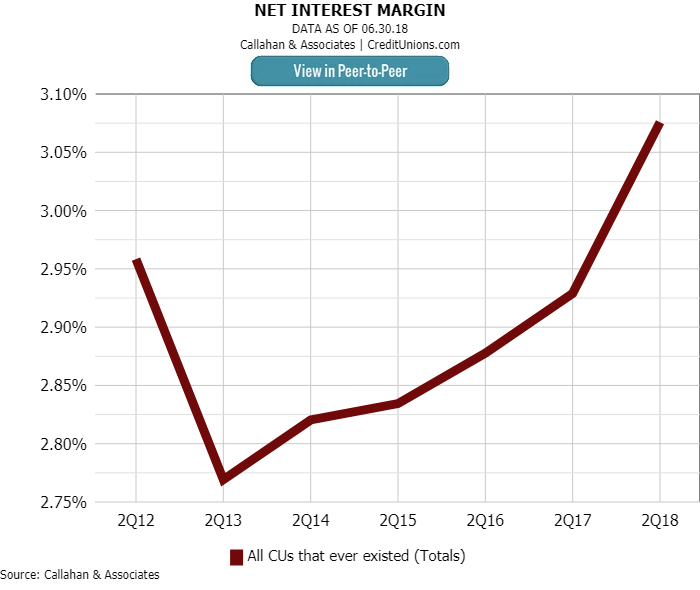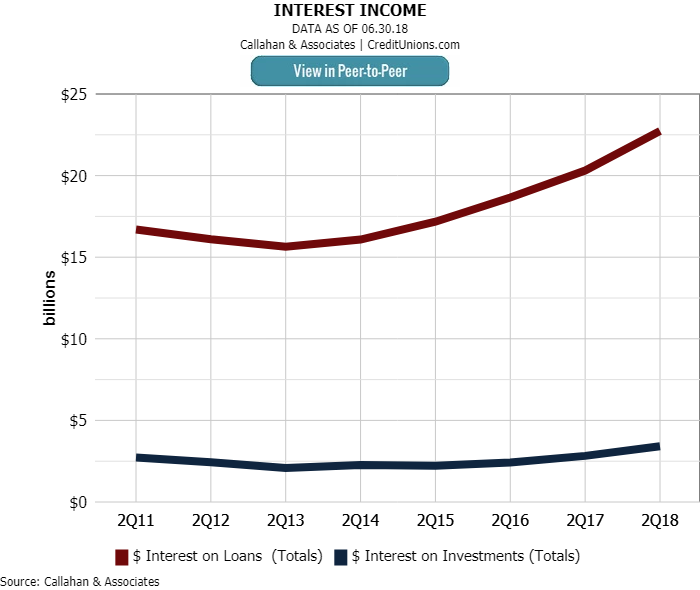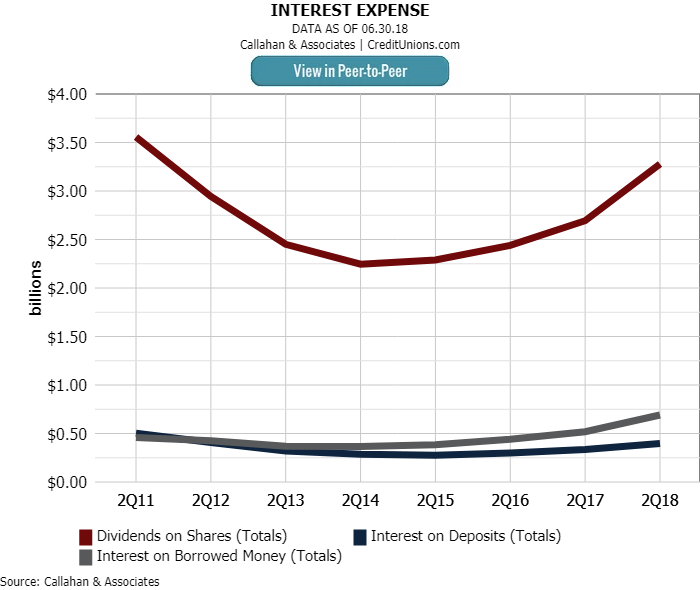# Interest Margin Expands For The Fifth Quarter

### The net interest margin at credit unions nationwide increased as interest income expanded more than \$3 billion in the past year.#### By Aman Johal

Nationally, the credit union net interest margin was up 13 basis points annually to 3.08% as of June 30, 2018. This is the highest level since year-end 2011 and marks the fifth consecutive quarter of increasing net interest margins, which is calculated by dividing the annualized difference between interest income and interest expense by average assets.

Among all U.S. credit unions, interest expenses increased 23.5% in the past 12 months, rising \$834.7 million to \$4.4 billion. Over the same period, interest income increased 13.4%, expanding more than \$3.0 billion to \$23.1 billion. With annual asset growth at 5.9% as of the second quarter, the jump in interest income resulted in an aggregate increase in the net interest margin for the industry.Net interest margins increased 13 basis points in the past year to 3.08% — the highest level since year-end 2011.

Interest on loans and interest on investments accounted for 86.9% and 13.1% of interest income, respectively. Credit unions recorded double-digit growth in both accounts over the past 12 months. Interest on loans grew 11.9% year-over-year and surpassed \$22.7 billion year-to-date as of June 30, 2018. Thats a \$2.4 billion increase in the past year. Interest on investments grew at a faster annual rate — 21.0%  and reached \$3.4 billion at midyear.Credit unions reported double-digit year-over-year growth in both interest on loans (11.9%) and interest on investments (21.0%).

Essentially three-quarters, 75.1%, of interest expense was paid in the form of dividends. At \$3.4 billion, dividend payouts increased 21.7% year-over-year. Interest on borrowed money, which is the fastest-growing interest expense, increased 33.2% year-over-year to \$690.6 million. Although interest expense grew at a faster rate, the relatively smaller dollar amount growth lead to an aggregate increase in the net interest margin.Dividends on shares increased 21.7% in the past year and accounted for 75.1% of total interest expense.

Aug. 20, 2018